SLVS861F August   2008  – June 2020

PRODUCTION DATA.

1. Features
2. Applications
3. Description
1.     Device Images
4. Revision History
5. Pin Configuration and Functions
6. Specifications
7. Detailed Description
1. 7.1 Overview
2. 7.2 Functional Block Diagram
3. 7.3 Feature Description
4. 7.4 Device Functional Modes
8. Application and Implementation
1. 8.1 Application Information
2. 8.2 Typical Application
1. 8.2.1 Design Requirements
2. 8.2.2 Detailed Design Procedure
3. 8.2.3 Application Curves
9. Power Supply Recommendations
10. 10Layout
11. 11Device and Documentation Support
1. 11.1 Device Support
2. 11.2 Documentation Support
3. 11.3 Related Links
5. 11.5 Electrostatic Discharge Caution
6. 11.6 Glossary
12. 12Mechanical, Packaging, and Orderable Information

• DGQ|10
• DGQ|10

#### 8.2.2.10 Error Amplifier Compensation

While current mode control typically requires only Type II compensation, it is desirable to layout for Type III compensation to increase flexibility during design and development.

Current mode control boost converters have higher gain with higher output impedance, so it is necessary to calculate the control loop gain at the maximum output impedance, estimated by Equation 57.

Equation 57.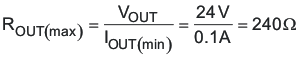The transconductance of the TPS40210-Q1 current-mode control can be estimated by Equation 58.

Equation 58.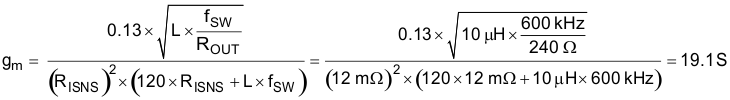The maximum output impedance, ZOUT, can be estimated by Equation 59.

Equation 59.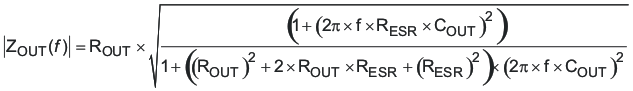Equation 60.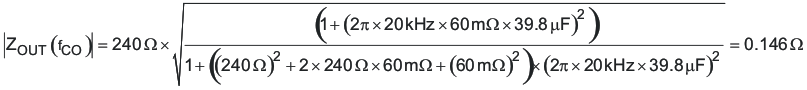The modulator gain at the desired cross-over can be estimated by Equation 61.

Equation 61.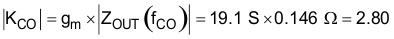The feedback compensation network needs to be designed to provide an inverse gain at the cross-over frequency for unit loop gain. This sets the compensation mid-band gain at a value calculated in Equation 62.

Equation 62.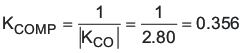To set the mid-band gain of the error amplifier to KCOMP, use Equation 63.

Equation 63.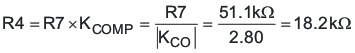R4 = 18.7 kΩ selected.

Place the zero at one 10th of the desired cross-over frequency.

Equation 64.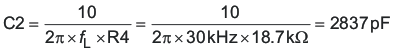C2 = 2200 pF selected.

Place a high-frequency pole at about five times the desired cross-over frequency and less than one-half the unity gain bandwidth of the error amplifier:

Equation 65.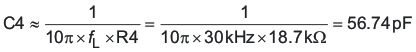Equation 66.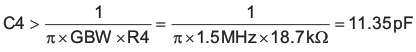C4 = 47 pF selected.